## A 20~\mu F20 μF capacitor has previously charged up to contain a total charge of Q = 100~\mu CQ=100 μC on it. The capacitor is then discharg

Question

A 20~\mu F20 μF capacitor has previously charged up to contain a total charge of Q = 100~\mu CQ=100 μC on it. The capacitor is then discharged by connecting it directly across a 100-k\Omega100−kΩ resistor. At what point in time after the resistor is connected will the capacitor have 13.5~\mu C13.5 μC of charge remaining on it?

in progress 0
5 months 2021-09-04T10:43:44+00:00 1 Answers 17 views 0

1. Explanation:

The given data is as follows.

C =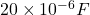R =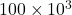ohm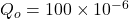C

Q =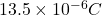Formula to calculate the time is as follows.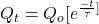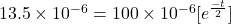0.135 =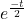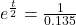= 7.407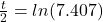t = 4.00 s

Therefore, we can conclude that time after the resistor is connected will the capacitor is 4.0 sec.